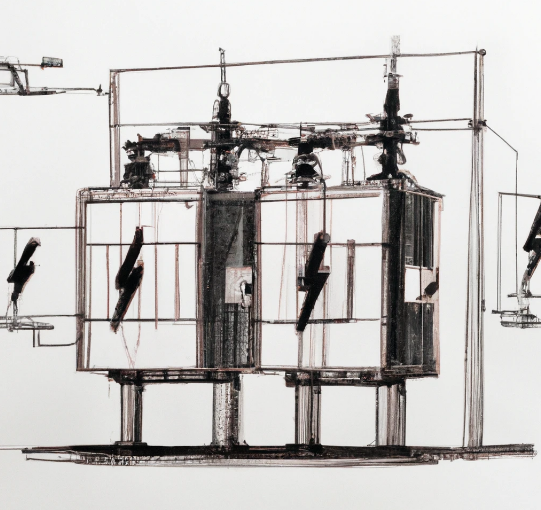## Transformer Secondary Fault Level

Last updated on 2023-04-03 2 mins. to read

## How to Calculate the Fault Level on the Secondary of a Three-Phase Transformer Using Percentage Impedance

Transformers are essential components in electrical power systems, transferring power between different voltage levels. One critical parameter to consider when designing and operating these systems is the fault level on the secondary side of a transformer. Knowing the fault level is essential for proper protective device selection and coordination.

The page will guide you through the process of calculating the fault level on the secondary side of a three-phase transformer using percentage impedance.

Step 1: Gather the necessary data

To calculate the fault level, you will need the following information:

1. Transformer's rated capacity (kVA)
2. Primary line-to-line voltage (V1)
3. Secondary line-to-line voltage (V2)
4. Transformer's percentage impedance (Z%)

Note: we will not be using the primary voltage, as we are assuming an infinite fault level on the transformer primary (network impedance = zero).The secondary side fault current (Isc) using this formula can be found using the following formula:

${I}_{b}=\frac{\mathrm{Transformer}\phantom{\rule{0.167em}{0ex}}\mathrm{kVA}×1000}{V2×\sqrt{3}×\left(\frac{Z%}{100}\right)}$        Ampere

The fault level on the secondary side in MVA is given by:

$\mathrm{Fault}\phantom{\rule{0.167em}{0ex}}\mathrm{Level}\left(\mathrm{MVA}\right)=\frac{I{\mathrm{sc}}_{}×V2×\sqrt{3}}{1,000,000}$

### Example Calculation

Let's go through an example calculation using the following data for a three-phase transformer:

• Transformer kVA: 1000 kVA
• Primary line-to-line voltage (V1): 11000 V
• Secondary line-to-line voltage (V2): 415 V
• Transformer percentage impedance (Z%): 6%

In this example, the fault level on the secondary side of the three-phase transformer is approximately 70.87 MVA.

### Online Calculator

If you need to calculate transformer secondary fault levels, you can use our online calculator. Using the calculator, you can determine the transformer's secondary three-phase fault current using an infinite or known fault level on the primary.